# James Meade facts for kids

Kids Encyclopedia Facts
Quick facts for kids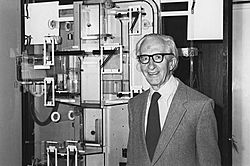Born 23 June 1907
Died 22 December 1995 (aged 88)
Nationality British
Institution London School of Economics
Field Macroeconomics
School or
Neo-Keynesian economics
Alma mater Oriel College, Oxford
Christ's College, Cambridge
Malvern College
Doctoral
students
Jacques Parizeau
Influences John Maynard Keynes
Contributions Theory of international trade and international capital movements
Awards Nobel Memorial Prize in Economic Sciences (1977)
Information at IDEAS / RePEc

James Edward Meade, CB, FBA (23 June 1907 – 22 December 1995) was a British economist and winner of the 1977 Nobel Memorial Prize in Economic Sciences jointly with the Swedish economist Bertil Ohlin for their "pathbreaking contribution to the theory of international trade and international capital movements".

Meade was born in Swanage, Dorset. He was educated at Malvern College and attended Oriel College, Oxford in 1926 to read Greats, but switched to Philosophy, Politics and Economics and gained an outstanding first. His interest in economics grew from an influential postgraduate year at Christ's College, Cambridge and Trinity College, Cambridge (1930–31), where he held frequent discussions with leading economists of the time including Dennis Robertson and John Maynard Keynes.

After working in the League of Nations and the Cabinet Office, he was the leading economist of the early years of Clement Attlee's government, before taking professorships at the London School of Economics (1947–1957) and the University of Cambridge (1957–1967).

## Early life and education

Meade was brought up in the city of Bath, Somerset in south-west England. He attended the Lambrook school in Berkshire from 1917 to 1921, where his education revolved around the Greek and Latin languages. In his time in Oriel College, Oxford, Meade switched at the end of his second year from Greats to Philosophy, Politics and Economics which was a very new concept at that time having started only in 1921. Meade's interest in economics grew due to various reasons.

He considered the heavy unemployment in the United Kingdom in the inter-war period as a menace and a social evil. His association with Major C. H. Douglas, to whom he was introduced to by his aunt, helped him come up with a cure for this evil.

In 1930 Meade was elected to a fellowship at Hertford College, Oxford. There he received the option of continuing his study of economics as a post graduate student. In 1930–31 Meade joined Trinity College, Cambridge after being invited to do so by Dennis Robertson whom he met through his great aunt.

While in Cambridge Meade became close friends with Richard Kahn, Piero Sraffa, Joan Robinson and Austin Robinson, forming the Cambridge Circus for economic discussion. Together they started discussing Keynes' work namely the A Treatise on Money. Every weekend Keynes appeared and was presented with the circle's discussion over the week by Kahn. They also discussed theories with Keynes when they met on Monday evenings at the political economy club in Keynes' room in King's College.

## Career

Meade became a lecturer at Hertford College, Oxford in 1931 and continued until 1937. Meade along with young enthusiasts such as Roy Harrod, Henry Phelps Brown, Charlie Hitch, Robert Hall, Lindley Fraser, Maurice Allen and Eric Hargreaves, who was his old tutor at Oriel College, started the concept of teaching economics as a regular subject for examination which was relatively new at Oxford. Meade was assigned with the responsibility of teaching the whole subject of economic theory. The economics of mass-unemployment and international economics interested Meade in particular. At that time Oxford had a really strong branch of the League of Nations Union with Gilbert Murray as its chairman and Margaret Wilson as its secretary. (Wilson married Meade in 1933.) Meade was made a member of the economics section of the League of Nations in Geneva in 1937. He worked as the main editor of the journal "World Economic Survey" and published the 17th and the 18th editions.

In April 1940 Meade was forced to leave Geneva for England with his family of three children because of the war. He became a member of the Economic Section of the War Cabinet Secretariat in England and remained member until 1947 rising to the post of Director in 1946. Meade was joined by Lionel Robbins and Keynes and together they used the section to solve everyday economic problems ranging from the rationing system to the pricing policy of nationalized companies. Meade became the professor of trade at London School of Economics in 1947 where the Economics department was headed by Lionel Robbins. While he was in Oxford, Meade had written a short textbook titled "An Introduction to Economic Analysis and Policy." Meade believed it was time to rewrite the book while teaching international economics, more precisely the theory of international economic policy. It slowly cultivated into Meade's two books, The Balance of Payments (1951) and Trade and Welfare (1955).

The first volume The Balance of Payments stresses the fact that for each of its policy objectives, the government requires a policy tool. The second volume Trade and Welfare deals with conditions under which free trade makes a country better off and conditions under which it does not. Meade concluded that, contrary to previous beliefs, if a country was already protecting one of its markets from international competition, further protection of another market could be "second best." That is, although the ideal would be to eliminate all trade barriers, if for some reason this was not feasible, then adding a carefully chosen dose of protectionism could improve the nation's economic well-being.

The two books took Meade a decade to complete, however according to him they still did not cover the entire field of international economic policy since he had given less attention to the issue of international aspects of economic growth or dynamic imbalance. Despite his words, Meade shared the Nobel Prize in Economics along with Bertil Ohlin in 1977.

In 1957 Meade moved from London to the chair of political economy in Cambridge, which he held until 1967, after which he resigned to become a senior research fellow of Christ's College, Cambridge. Meade left the fellowship at the retirement age in 1974. During this time Meade started thinking about writing one or two volumes on the domestic aspects of economic theory and policy. He successfully wrote four volumes in this series namely The Stationary Economy, The Growing Economy, The Controlled Economy, and The Just Economy. Even after the four volumes Meade still believed that he had just made the beginning. He believed that the frontiers of knowledge when it comes to economics keep expanding at such a rate that it was almost impossible to establish a soundly based understanding of the entire subject and its ever-evolving parts.

In 1974 Meade took time off to act as full-time chairman of a committee set up by the Institute for Fiscal Studies to examine the structure of direct taxation in the United Kingdom. The committee consisted of a number of first-rate economic theorists and of leading practitioners in tax law, accountancy and administration.

In 1976, he was awarded an Honorary Degree (Doctor of Science) by the University of Bath.

Meade died on 22 December 1995 in Little Shelford, Cambridgeshire.

## Meade's model of economic growth

The basic assumptions for J.E.Meade's model are as follows: (1) The economy in question is a closed economy with no relationship with the outside world. (2) There is no government activity involving taxation and expenditure. (3) Perfect competition exists in the market. (4) Constant returns to scale prevails in the economy. (5) There are only two commodities-a consumption good and a capital good. (6) There is full employment of land, labour and machinery. (7) All machinery are alike and the ratio of labour to machinery can be easily varied, hence there is perfect malleability of machinery. (8) There is perfect substitutability between capital goods, consumption goods and any given stock of machines, no matter how old or new they are, a certain percentage gets replaced every year. Meade calls this phenomenon the assumption of depreciation by evaporation.

### Determinants of the rate of economic growth

According to the assumptions given above, the net output produced by the economy depends on the following four things: (1) The amount of existing stock of machines in the economy (2) The amount of labor for production process (3) The amount of land or natural resources available for productive use in the economy (4) The technological progress in the economy which is expected to improve over time.

Hence the production function for the economy is given by: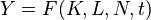$Y = F (K,L,N,t)$

Where: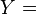$Y =$ net output or net real income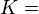$K =$ existing stock of machines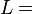$L =$  the amount of labour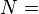$N =$ amount of land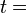$t =$  time


Time is accounted for because with the passage of time the production would increase without any increase in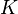$K$,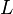$L$, or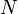$N$. An increase in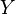$Y$ with time (denoted by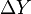$\Delta Y$) can take place in three ways. First, the machine stockpile may increase if the community starts saving part of their income thereby accumulating real capital. If the increase in the stock of capital taking place in one year is given by$\Delta Y$, the output would increase by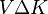$V \Delta K$ where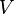$V$ denotes the marginal net physical product of a machine.

Secondly,$L$, the working population, may grow. If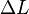$\Delta L$ denotes an increase in the amount of labour productivity employed in a single year and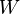$W$ measures the marginal product of labour, the output will increase in that year by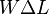$W \Delta L$.

Finally, the net output can increase if there is an increase in the technical progress (hence enabling increased efficiency). The total increase in net output due to technical progress is given by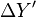$\Delta Y'$. Hence the total increase in net output in one year is the sum of the three influences. Combining this we get the equation: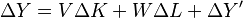$\Delta Y = V \Delta K + W \Delta L + \Delta Y'$

Dividing both sides by$Y$, we get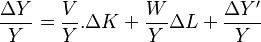$\frac{\Delta Y}{Y} = \frac{V}{Y} . \Delta K + \frac{W}{Y} \Delta L + \frac{\Delta Y'}{Y}$

Or,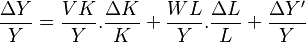$\frac{\Delta Y}{Y} = \frac{VK}{Y} . \frac{\Delta K}{K} + \frac{WL}{Y} . \frac{\Delta L}{L} + \frac{\Delta Y'}{Y}$ (Equation 1)

Here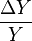$\frac{\Delta Y}{Y}$ is the annual proportionate rate of growth of output,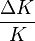$\frac{\Delta K}{K}$ the annual proportionate rate of growth of machinery stock,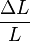$\frac{\Delta L}{L}$ the annual proportionate rate of growth of productively employed labour and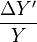$\frac{\Delta Y'}{Y}$ the annual proportionate rate of growth of output due solely to increase in technical progress.

Meade denotes these four proportionate rates of growth as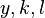$y , k , l$ and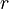$r$ respectively.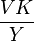$\frac{VK}{Y}$ is the proportion of net national income to be paid in net profits (provided the owners of machinery receive a reward equal to the value of the net marginal product of the machinery). Meade denotes this as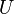$U$ and calls it "the proportional marginal product of machinery". Under the assumption of constant returns to scale, it is equal to the proportion of national income received in profits. Similarly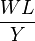$\frac{WL}{Y}$ represents the proportional marginal product of labour and is equal to the proportion of the net national income going to wages under conditions of constant-returns competitive equilibrium. Meade denotes this as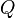$Q$. Hence equation 1 can be written as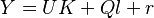$Y = UK +Ql + r$ (Equation 2)

This shows the growth rate of output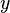$y$ as being the weighted sum of three other growth rates, the sum of the growth rate in the stock of machines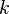$k$ weighted by the marginal importance of machinery in the productive process i.e., in a competitive equilibrium by the proportion of the national income going to profits$U$ plus the growth rate of the population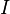$I$ weighted by the marginal importance of labour in the productive process or, in a competitive equilibrium by the proportion of income going to wages$Q$ plus the growth rate of technical progress$r$ Hence equation 2 can be written as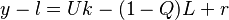$y - l = Uk - (1 - Q)L + r$ (Equation 3)

Since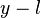$y - l$ is the difference between the growth rate of total output and growth rate of the workforce, the growth rate of the real income per head can be measured. For example, if the total real income is increasing by 10 percent every annum but the working population is growing at 8 percent per annum, the income per head is rising by approximately 2 percent per annum.

Equation 3 shows that the growth rate of real income per head (y – l) is the output of three factors.

Firstly it is raised by the growth rate in real capital$k$ weighted by its proportional marginal product or by the proportion of net national income which would be paid min profits in a competitive equilibrium$U$. Secondly it is depressed by the growth rate in the working population$l$ weighted by one minus the proportional marginal product of labour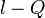$l - Q$. Lastly it is raised by the amount of technology in the economy$r$.

The element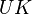$UK$ in equation 3 can also be written down as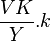$\frac{VK}{Y} . k$ since the growth rate of machine stock is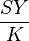$\frac{SY}{K}$ where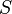$S$ is the proportion of the net national income that is saved. Therefore, we have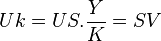$Uk = US . \frac{Y}{K} = SV$ which expresses the same thing in three forms namely the contribution which capital accumulation makes to the growth rate of the final output. Hence the basic relationship between the growth rate of real income per head and its three basic determinants can be expressed as: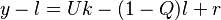$y - l = Uk - (1 - Q)l + r$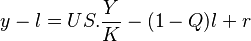$y - l = US . \frac{Y}{K} - (1 - Q)l + r$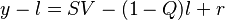$y - l = SV - (1 - Q)l + r$

Meade explains the application of these equations by taking a simple numerical example. Suppose the people save one tenth of their income such that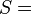$S =$ and that the marginal product of real capital goods or the market profit rate is 5 percent per annum. Hence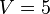$V = 5$ percent per annum. The contribution of capital accumulation to the growth of output,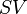$SV$ would be one tenth of 5 percent per annum. Hence ½ percent per annum. The explanation of this is, out of a year's income of 1000, if people save 100 units of product and if a once-for-all addition of 100 units to the stock of machines increases annual output in every future year by 5 units, then the initial annual income of 1000 will be raised by this year's capital accumulation to 1005 or by ½ percent during the course of the year. Assuming initial annual income to be 1000 and the initial machinery stock to be 2000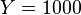$Y = 1000$ and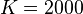$K = 2000$. Similarly, the same thing can be expressed by saying that the stock of machines had increased from 2000 to 2100 or by 5 percent per annum. Then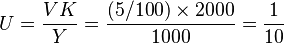$U = \frac{VK}{Y} = \frac{(5/100) \times 2000}{1000} = \frac{1}{10}$ and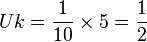$Uk = \frac{1}{10} \times 5 = \frac{1}{2}$ per annum.

Thus the contribution of capital accumulation to growth rate of the final output was one tenth of 5 percent per year or ½ percent per annum.

The same result can be obtained by multiplying the proportion of the national income going to profits$U$, the proportion of the national income which is saved$S$ and the annual income to capital stock ratio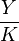$\frac{Y}{K}$ Our numerical example is: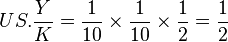$US . \frac{Y}{K} = \frac{1}{10} \times \frac{1}{10} \times \frac{1}{2} = \frac{1}{2}$ percent per annum.

### Critical appraisal of Meade's growth theory

The growth theory provided by Meade is neo-Classical in nature. It is simple and attractive as it promises a state of steady economic growth. However it suffers from a few drawbacks First, for a steady state of economic growth to exist, the technological progress should be assumed to be entirely labour-augmenting. This is the special case of Harrod-neutral technological progress. However this does not seem to exist in the model.

Second, the neo-Classical adjustment mechanism depends on the flexibility of factor prices. But if they are not flexible a lot of difficulty is established. For example, in the Keynesian liquidity trap, the interest rate may fail to go down beyond a minimum level hence preventing the capital-output ratio to be high enough to reach growth equilibrium.

Third, there is no mention about investment function in the model. It is assumed to be solely determined by savings. Hence entrepreneurial expectations about the future are not taken into account.

Fourth, in the neo-Classical models capital is assumed to be jelly-like, homogeneous and malleable. This assumption is truly unrealistic, but without it present, it becomes very difficult to reach a stage of steady growth.

Finally, the technological progress is considered to be totally exogenous which is again extremely unrealistic and has been pointed out by many economists. To sum up, the neo-Classical growth model of Meade is based on certain restrictive and unrealistic assumptions. Hence applying this model in the case of under developed nations is almost impossible since the assumptions of perfect competition, full employment of labour and machinery and constant returns to scale do not fit in their economic realities.

## Other contributions

Professor Meade made other contributions to economics. For example, he showed that the labour-managed firm (or worker cooperatives) need not respond inefficiently to price rises even in theory. Along with fellow Neo-Keynesian economist James Tobin in 1980, Meade proposed nominal GDP targeting as a monetary policy rule during his Nobel Prize memorial lecture on 8 December 1977.

## Published works

His books include:

• The Theory of International Economic Policy – The Balance of Payments (1951)
• The Theory of International Economic Policy – Trade and Welfare (1955)
• Principles of Political Economy (1965–76)
• The Intelligent Radical's Guide To Economic Policy (1975)
• Liberty, Equality and Efficiency (1993)James Meade Facts for Kids. Kiddle Encyclopedia.